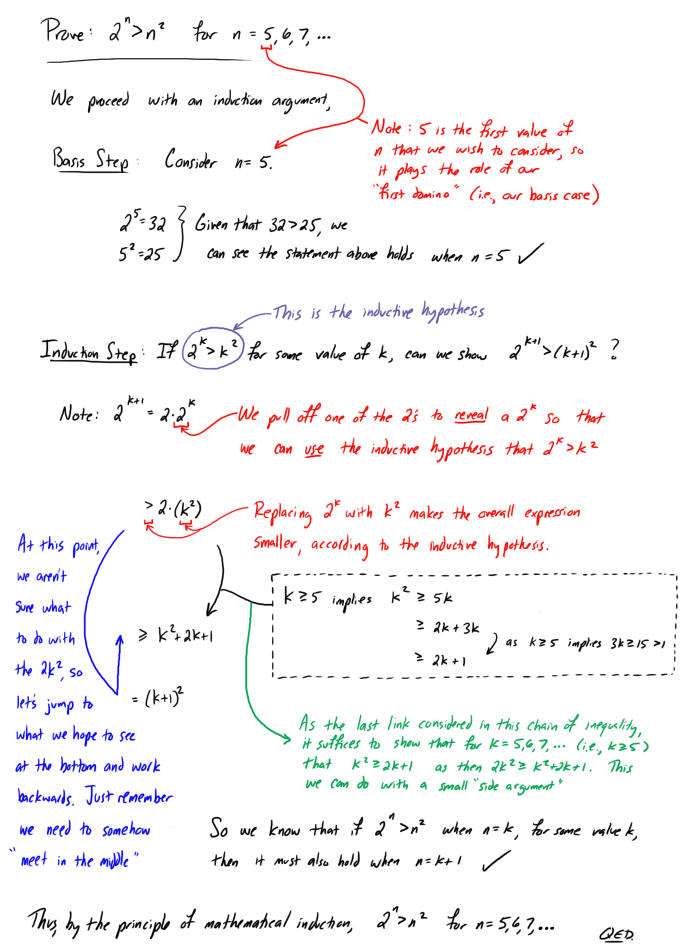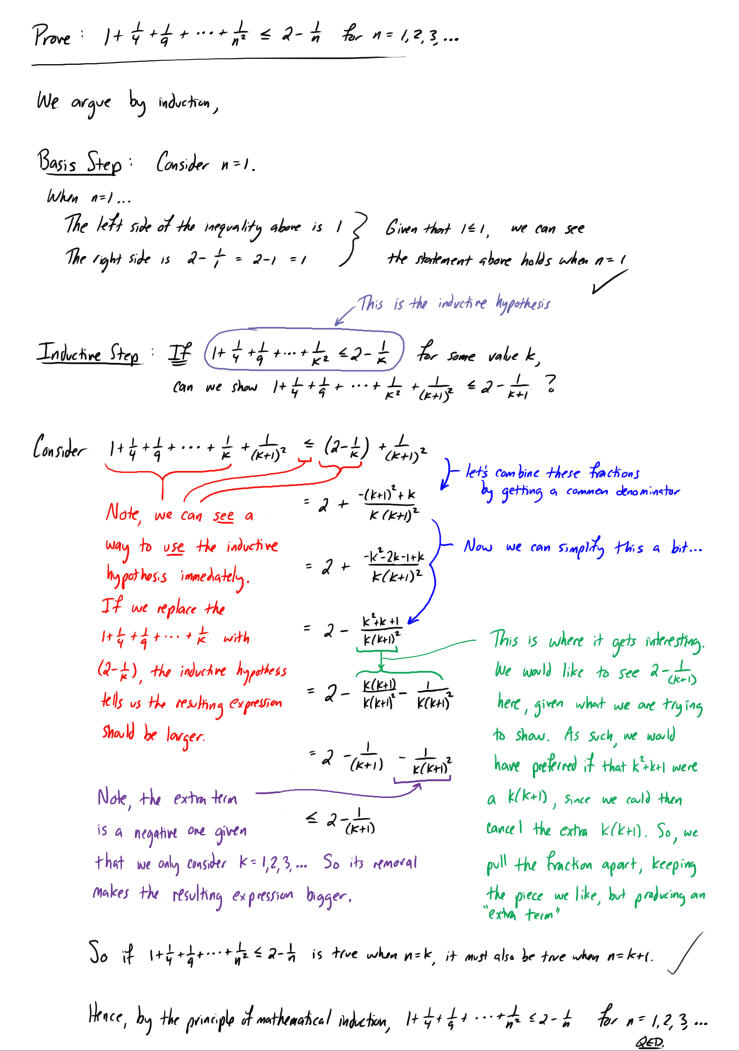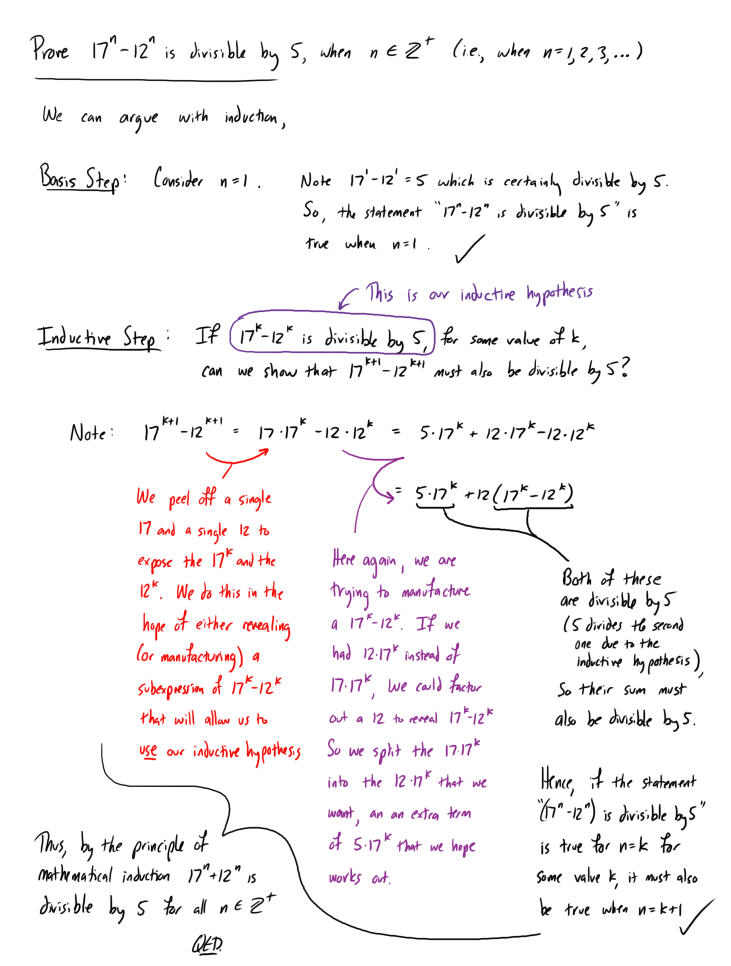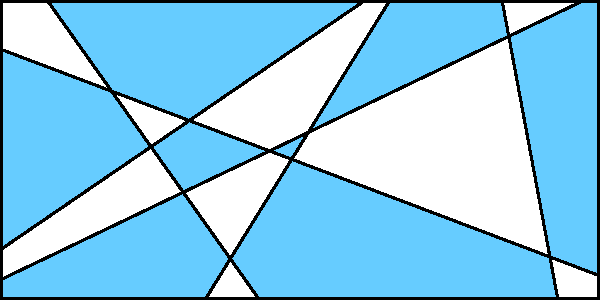Exercises - Induction in Other Contexts

$$\require{AMSsymbols}$$
1. Use mathematical induction to prove the given inequalities are true for the indicated values of $n$.

1. Let us argue by induction...

Starting with the basis step, we must show that the statement holds for the smallest value of $n$ that we wish to consider.

Since we seek to prove the statement holds for every positive integer, then the smallest value we wish to consider would be $n=1$.

When $n=1$, the statement reduces to $3^1 > 2 \cdot 1$, which of course, is true.

Now we proceed with the inductive step, where we must show that if we know the statement holds for some particular value of $n$, say $n=k$, then the statement must also hold when $n=k+1$.

Equivalently, for this problem, we need to show that if the following "inductive hypothesis" is true for some particular value of $k$ $$3^k > 2k$$ then, it must also be true that $$3^{k+1} > 2(k+1)$$

To demonstrate this last inequality, we will attempt to create a string of equalities/inequalities that starts at $3^{k+1}$ and terminates at $2(k+1)$, like the following: $$3^{k+1} = \cdots > \cdots = \cdots \geq \cdots = 2(k+1)$$ Note: as long as there is one strict inequality symbol in the string that we generate, we will be able to conclude $3^{k+1} > 2(k+1)$.

To begin, let us separate a factor of $3$ from $3^{k+1}$ to give us an opportunity to use our inductive hypothesis (which is a statement about $3^k$):

$$3^{k+1} = 3 \cdot 3^k \quad \cdots \quad ? \quad \cdots \quad 2(k+1)$$

Our inductive hypothesis assures us that $3^k > 2k$, so

$$3^{k+1} = 3 \cdot 3^k > 3 \cdot (2k) \quad \cdots \quad ? \quad \cdots \quad 2(k+1)$$

$$3^{k+1} = 3 \cdot 3^k > 3 \cdot (2k) = 6k \quad \cdots \quad ? \quad \cdots \quad 2k+2 = 2(k+1)$$

If we knew $6k \geq 2k+2$, we would be done, right? This, fortunately, can be quickly deduced from the fact that $k$ is a particular value of $n$, and the only values of $n$ we are considering are positive integers. Consequently,

$$\begin{array}{rcl} k \geq 1 &\Rightarrow& 2k \geq 1\\\\ &\Rightarrow& 4k \geq 2\\\\ &\Rightarrow& 6k \geq 2k+2 \end{array}$$

So,

$$3^{k+1} = 3 \cdot 3^k > 3 \cdot (2k) = 6k \geq 2k+2 = 2(k+1)$$

and hence,

$$3^{k+1} > 2(k+1)$$

which is what we needed to show.

Having met with success in both the basis and inductive steps, we are free to conclude by the principle of mathematical induction, that the following is true for every positive integer $n$

$$3^n > 2n$$ QED.

Here is another, more elegant way to demonstrate the inductive step above:

Recall that $k$ is a particular value of $n$, and the only values of $n$ that are being considered are positive integers, so $k \geq 1$. As such,

$$3^k > 1$$

But then,

$$2 \cdot 3^k > 2$$

Now, adding this inequality to our inductive hypothesis

$$3^k > 2k$$ we arrive at

$$3 \cdot 3^k > 2k + 2$$

or equivalently,

$$3^{k+1} > 2(k+1)$$

which is what was needed to show to complete the inductive step

$\ddot\smile$

2.3.4.5. $\displaystyle{(1+x)^n \ge 1 + nx}$; $n=1,2,3,\ldots$

2. Use mathematical induction to prove that if $n \in {\Bbb Z^+}$, then the following statements are true.

1.2. $6^n + 4$ is divisible by $5$

3. $5^n + 2(11^n)$ is divisible by $3$

4. $4^n + 5^n + 6^n$ is divisible by $15$ when $n$ is a positive odd integer

5. $3 \mid 2^{2n} - 1$

6. There exist distinct integers $x$, $y$, and $z$ for which $x^2 + y^2 + z^2 = 14^n$
(Hint: you might want to consider even n and odd n separately.)

7. Suppose we proceed to argue by induction (although there are more efficient approaches, as the bottom of this page indicates).

Starting with the basis step, we must show that the statement holds for the smallest value of $n$ that we wish to consider.

Since we seek to prove the statement holds for every positive integer, then the smallest value we wish to consider would be $n=1$.

When $n=1$, we note $n^3+n = 1^3 + 1 = 2$, which is even. Thus, the statement is true when $n=1$.

Now we proceed with the inductive step, where we must show that if we know the statement holds for some particular value of $n$, say $n=k$, then the statement must also hold when $n=k+1$.

Equivalently, for this problem, we need to show if the following "inductive hypothesis" is true for some particular value $k$, $$k^3 + k \textrm{ is even}$$ then it must also be true that $$(k+1)^3 + (k+1) \textrm{ is even}$$

To this end, we start by expanding this last expression and regrouping the resulting terms, so that we might create an opportunity to use the inductive hypothesis: $$\begin{array}{rcl} (k+1)^3 + (k+1) &=& k^3 + 3k^2 + 3k + 1 + k + 1\\\\ &=& (k^3 + k) + 3k^2 + 3k + 2\\\\ &=& (k^3 + k) + 3k(k+1) + 2\\\\ \end{array}$$

Note, $3k(k+1)$ must be even as either $k$ or $(k+1)$ must be even. Since $(k^3+k)$ was assumed even (by our inductive hypothesis), and 2 is obviously even as well, we have expressed $(k+1)^3 + (k+1)$ as the sum of three even values. As such $(k+1)^3 + (k+1)$ must be even -- which is what we needed to show.

Having met with success in both the basis and inductive steps, we are free to conclude by the principle of mathematical induction, that $n^3 + n$ must be even for every positive integer $n$.

QED.

As suggested above, however, we can argue the same conclusion much more efficiently with a different (non-induction based) strategy:

Suppose we argue by cases:

We know that every positive integer $n$ must be either even, or odd, so

1. If $n$ is even, then $n^3$ must also be even. So $n^3 + n$ is the sum of two even values, which must be even.

2. If $n$ is odd, then $n^3$ must also be odd. So $n^3 + n$ is the sum of two odd values, which also must be even.

As such, for every positive integer $n$, it must be true that $n^3 + n$ is even.

QED.

3. Prove that if $n$ is an integer greater than or equal to 2, and the below radical contains exactly $n$ ones, it must be irrational.

Prove that if $n$ is an integer greater than or equal to 2, and the below radical contains exactly $n$ ones, it must be irrational.

$$\sqrt{1 + \sqrt{1 + \sqrt{1 + \sqrt{1 + \cdots}}}}$$

We can argue by induction...

First, we establish the basis step. When $n=2$, the number in question is $\sqrt{1+\sqrt{1}} = \sqrt{2}$, whose irrationality is well-known.

Now we proceed with the inductive step. We need to show that if the expression above is irrational when their are $k$ ones, then it must also be irrational when there are $k+1$ ones.

Let us argue the inductive step indirectly. Suppose

$$\underbrace{\sqrt{1 + \sqrt{1 + \sqrt{1 + \sqrt{1 + \cdots}}}}}_{k+1 \textrm{ ones}} = r \quad , \quad r \in \mathbb{Q}$$

Squaring, and then subtracting $1$ from both sides, we arrive at

$$\underbrace{\sqrt{1 + \sqrt{1 + \sqrt{1 + \sqrt{1 + \cdots}}}}}_{k \textrm{ ones}} = r^2 - 1 \quad , \quad r \in \mathbb{Q}$$

However, the rationals are closed under multiplication and subtraction, so $r^2 - 1$ must itself be rational.

As such, the expression on the left must be rational. This, of course, contradicts our assumption of its irrationality. Having reached a contradiction, it must be the case that our assumption is false, and its reverse true:

$$\underbrace{\sqrt{1 + \sqrt{1 + \sqrt{1 + \sqrt{1 + \cdots}}}}}_{k+1 \textrm{ ones}} \in \mathbb{Q}$$

This is what we needed to show to complete the inductive step of our argument, so by the principle of mathematical induction, if $n$ is an integer greater than or equal to 2, and the below radical contains exactly $n$ ones, it must be irrational.

$$\sqrt{1 + \sqrt{1 + \sqrt{1 + \sqrt{1 + \cdots}}}}$$ QED.

4. Use mathematical induction to prove that for $n>23$, there exists non-negative integers $x$ and $y$ such that $n=7x+5y$.

We shall argue by cases, proving each case with induction...

We have $5$ cases: $n$ could have remainder $0$, $1$, $2$, $3$, or $4$ upon division by $5$.

Establishing the basis step for cases involving remainders of $4$, $0$, $1$, $2$, and $3$ (in that order), we observe:

• $24 = 7 \cdot 2 + 5 \cdot 2$
• $25 = 7 \cdot 0 + 5 \cdot 5$
• $26 = 7 \cdot 3 + 5 \cdot 1$
• $27 = 7 \cdot 1 + 5 \cdot 4$
• $28 = 7 \cdot 4 + 5 \cdot 0$

To argue the inductive step for each case, notice that if the statement is true for some $n=k$, then $k=7x+5y$, which in turn implies $k+5 = 7x + 5(y+1)$. As $n=k+5$ is the next value of $n$ with the same remainder as $k$, the inductive step is established.

Therefore, by the principle of mathematical induction, for $n>23$, there must always exist non-negative integers $x$ and $y$ such that $n=7x+5y$.

QED.

5. Consider any finite collection of straight lines in a plane. Prove that the regions in the plane created by these lines can be colored in such a way that any two regions sharing a common edge have different colors, as suggested by the following image.6. 🔎 Suppose you have three $8 \times 8$ chessboards, each with a single square missing. The first is missing one of the corner squares. The second is missing a square in its second column, eighth row. The third is missing one of the four center squares. Demonstrate that it is possible to cover the remaining squares of each board with L-shaped pieces, where each L-shaped piece covers three squares. Given a $2^n \times 2^n$ chessboard that is missing one square, is it always possible to cover the remaining squares of the chessboard with L-shaped pieces? Prove this claim, or provide a counter-example.

7. 🔎 A platonic solid is defined to be a regular, convex polyhedron whose faces are congruent, regular polygons, with the same number of faces meeting at each vertex. Prove that there are only five such platonic solids (i.e., tetrahedron, cube, octahedron, dodecahedron, and icosahedron) under the assumption that $V - E + F = 2$, where $V$ counts the number of vertices, $E$ counts the number of edges, and $F$ counts the number of faces of the polyhedron.

8. We shall argue using the second principle of mathematical induction (i.e., the strong principle of mathematical induction)...

First, we establish the basis step. Consider a puzzle that consists of only $1$ piece. Such a puzzle is already "solved", so zero moves are required. As $n-1=0$, the basis step is established.

Now we turn our attention to the inductive step. Using strong induction, we need to show that if the statement holds whenever $n \le k$, then it must also hold when $n=k+1$.

Consider the last move that solves a puzzle of $k+1$ pieces. Suppose that, during this last move the blocks joined were named $A$ and $B$, and had $a$ and $b$ pieces respectively. Note, $a+b = k+1$ and $a,b \le k$. As such by the inductive hypothesis, block $A$ could be put together in $a-1$ moves and block $B$ could be put together in $b-1$ moves. Thus, the entire puzzle can be put together in $(a-1) + (b-1) + 1 = a + b - 1 = k$ moves -- which is what we needed to show.

Hence, by the strong principle of mathematical induction, exactly $n-1$ moves are required to solve a jigsaw puzzle with $n$ pieces.

QED.

9. 🔎 Recall the rules of the game of Chomp. Played by two players (player $A$ who goes first, and player $B$ who goes second) one starts with a rectangle of chocolate, whose upper left square is poisoned. The game proceeds in turns, where on each player's turn, he or she chooses one square of the chocolate and eats that square as well as all the squares below and to the right of it (including any squares directly below or to the right). The player who eats the poisoned square loses.

Below shows a sequence of moves in a typical game, starting with a $3 \times 5$ bar:In the last move, player $A$ must eat the last (poisoned) block and so loses.

One obviously wants to develop a strategy for avoiding being poisoned. Prove that one of the two players must have a winning strategy. That is to say -- for any given board -- one of the players, if they play perfectly, can win every time no matter what choices the other player makes.

10. Consider the first several values of $f(n)$,

 $n$ 1 2 3 4 5 6 7 8 9 10 $f(n)$ 1 5 7 17 31 65 127 257 511 1025

It appears that $f(n) = 2^n + (-1)^n$. Let us show this by the second principle of induction (i.e., strong induction).

The above table verifies the basis step, so it remains to consider the inductive step.

Using strong induction, we need to show that if $f(n) = 2^n + (-1)^n$ for all $n \le k$ for some integer $k$, then $f(k+1) = 2^{k+1} + (-1)^{k+1}$.

At this point the argument is direct:

$$\begin{array}{rcl} f(k+1) &=& f(k) + 2 f(k-1)\\ &=& \left[ 2^k + (-1)^k \right] + 2 \cdot \left[ 2^{k-1} + (-1)^{k-1}\right]\\ &=& 2^k + (-1)^k + 2^k - 2(-1)^k\\ &=& 2 \cdot 2^k - (-1)^k\\ &=& 2^{k+1} + (-1)^{k+1} \end{array}$$

Hence, by the second principle of mathematical induction, $f(n) = 2^n + (-1)^n$ for all positive integers $n$.

11. The tower of Hanoi was a popular puzzle of the late nineteenth century. The puzzle includes three pegs and some number of rings of different sizes placed in order of size, with the largest on the bottom, on one of the pegs. The goal of the puzzle is to move all the rings, one at a time without ever placing a larger ring on top of a smaller ring, from the first peg to the second, using the third peg as an auxiliary peg.

12. 🔎 Suppose a sequence $x_1,x_2,\ldots$ is defined by $x_1 = 5$, $x_2 = 13$, and $x_{n+1}=5x_n - 6x_{n-1}$ for $n \ge 2$. Prove that $$x_n = 2^n + 3^n$$

13. We argue indirectly. Assume that there does not exist any positive integer $n$ such that $na \ge b$. That is to say, assume $$na < b \quad \forall n \in \mathbb{Z}^+$$

Subtracting $na$ from both sides, we then have $$b - na > 0 \quad \forall n \in \mathbb{Z}^+$$ This gives us a large collection of non-negative values with which to work -- perhaps the well-ordering principle might be useful here. Suppose we construct the set $S$ made up of all of these values. That is to say, suppose $$S = \{b - na, \textrm{ where } n \in \mathbb{Z}^+ \}$$ $S$ is clearly a non-empty set of non-negative values, so the well-ordering principle guarantees it has a least element, say $m = b - n_m a$.

But what happens if we consider $x = b - (n_m+1) a$?

Certainly, by its construction $x \in S$ and $x \lt m$. This contradicts $m$ being the least element of $S$.

Having reached a contradiction, the opposite of our assumption must be true. There must indeed exist some positive integer $n$ such that $na \ge b$.

QED.

14. Show that for any integer $n \ge 0$ we have $$\int_0^{\infty} x^n e^{-x} dx = n!$$

15. Prove that for every integer $n \ge 1$, $$\frac{d^n}{dx^n} \left[ xe^{2x} \right] = 2^{n-1}(2x+n)e^{2x}$$

16. Let $a_1, a_2, \ldots$ be a sequence of real numbers satisfying $a_{i+j} \le a_i + a_j$ for all positive integers $i$ and $j$. Use the principle of strong induction to prove:

$$a_n \le a_1 + \frac{a_2}{2} + \frac{a_3}{3} + \cdots + \frac{a_n}{n}$$

Can we argue with induction? Note, strong induction allows us to assume more...

Let us argue by strong induction...

Clearly, the inequality holds for $n=1$

We need to show that if it holds for $n = 1, 2, 3, \ldots, k$, then it must also hold for $n=k+1$

What can we determine immediately? In particular, what can we conclude from the given nature of the sequence $\{a_n\}$?

Starting with the given fact that $a_{i+j} \le a_i + a_j$ for all positive integers $i$ and $j$, and the corresponding implications for $a_{k+1}$, we notice

$$\begin{array}{rcl} a_{k+1} &\le& a_1 + a_k\\ a_{k+1} &\le& a_2 + a_{k-1}\\ a_{k+1} &\le& a_3 + a_{k-2}\\ &\vdots&\\ a_{k+1} &\le& a_k + a_1 \end{array}$$

$$ka_{k+1} \le 2(a_1 + a_2 + a_3 + \cdots + a_k)$$

How can we use the inductive hypothesis? Can we relate it to the inequality above, for example...

Now, turning our attention to the inductive hypothesis, we know that:

$$\begin{array}{rcl} a_1 &\le& a_1\\\\ a_2 &\le& a_1 + \frac{a_2}{2}\\\\ a_3 &\le& a_1 + \frac{a_2}{2} + \frac{a_3}{3}\\\\ &\vdots&\\\\ a_k &\le& a_1 + \frac{a_2}{2} + \frac{a_3}{3} + \cdots + \frac{a_k}{k} \end{array}$$

Adding these together, we can get an inequality that also involves the expression $a_1 + a_2 + a_3 + \cdots + a_k$.

$$a_1 + a_2 + a_3 + \cdots + a_k \le ka_1 + (k-1) \frac{a_2}{2} + (k-2) \frac{a_3}{3} + \cdots + \frac{a_k}{k}$$

Can we insert what we would like to see, and then compensate for that insertion? The other inequality involved twice the sum...

Rather than simply doubling both sides to achieve linkage with the earlier inequality deduced, we instead add $a_1 + a_2 + \cdots + a_k$ to both sides to the same end. This has the advantage that, upon collecting like terms on the right, the right side starts to look more recognizable...

$$2(a_1 + a_2 + a_3 + \cdots + a_k) \le (k+1) \left( a_1 + \frac{a_2}{2} + \cdots + \frac{a_k}{k} \right)$$

How might we combine what we know? (i.e., the two inequalities)

Combining our two results above, we have

$$ka_{k+1} \le (k+1) \left( a_1 + \frac{a_2}{2} + \cdots + \frac{a_k}{k} \right)$$

Can we insert what we want and compensate for the insertion? We seem to be missing the $\frac{a_{k+1}}{k+1}$ term...

Recalling that the inequality we are trying to prove has an additional $\frac{a_{k+1}}{k+1}$ term, we add $a_{k+1}$ to both sides. It follows that

$$(k+1) a_{k+1} \le (k+1) \left( a_1 + \frac{a_2}{2} + \cdots + \frac{a_k}{k} + \frac{a_{k+1}}{k+1} \right)$$

Can we simplify things? We have a common (positive) factor on both sides...

Finally, dividing by $(k+1)$ above yields the desired result

$$a_{k+1} \le a_1 + \frac{a_2}{2} + \cdots + \frac{a_k}{k} + \frac{a_{k+1}}{k+1}$$